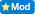How much farther does a golfball fly on the equator going east versus going west?

0  Views: 807 Answers: 2 Posted: 7 years ago

Is this a science question or are you just trying to find a good tee off spot?     :))

P.S.   I'm a (sort of) golfer. I can relate.

Benthere

....I bet you are attracted to the water hazards !
DuckyYes. I'm Aquarius. :)

The centrifugal force (which points away from the rotational axis) is m*r*w^2 , where R is the radius of the earth, and w is the angular acceleration of the earth. At the equator, this points directly vertically at the surface, so all it does is make a tiny correction to gravity. we'll call that corrected value g (it'll actually be the gravitational g minus r*w^2).

The coriolis force, at the equator, is zero... so it simplifies the discussion somewhat. (The coriolis force depends as the cosine of the angle from the north pole. It is maximum at the poles, zero at the equator, and in opposite directions on the northern and southern hemispheres).

So, all we have is that gravity is slightly reduced. What will be the effect? Look at the formulae for projectile motion, both the maximum height and range are proportional to 1/g... so if g is reduced, both will be increased. R*w^2 is 3.39 x 10^-2, which is quite a bit smaller than g. The correction is therefore very small, about 0.3 % of g.

I don't think the rotation of the earth has any effect like the one you asked about, that is, if you throw a ball in the direction opposite to the earth's rotation, the distance traveled won't be increased. Why? For the same reason that a ball throw vertically from a moving car lands in the car again. Think about it. http://www.physicsforums.com/archive/index.php/t-218020.html

:)

fusionrudy

Why then is there such a huge difference weather you launch a satellite east or west?
ColleenI didn't write it. Click the link at the bottom of my post. It will take you to the forum where this was discussed.

ROMOS
Karma: 2940

Bob/PKB
Karma: 1830

Colleen
Karma: 1760

Benthere